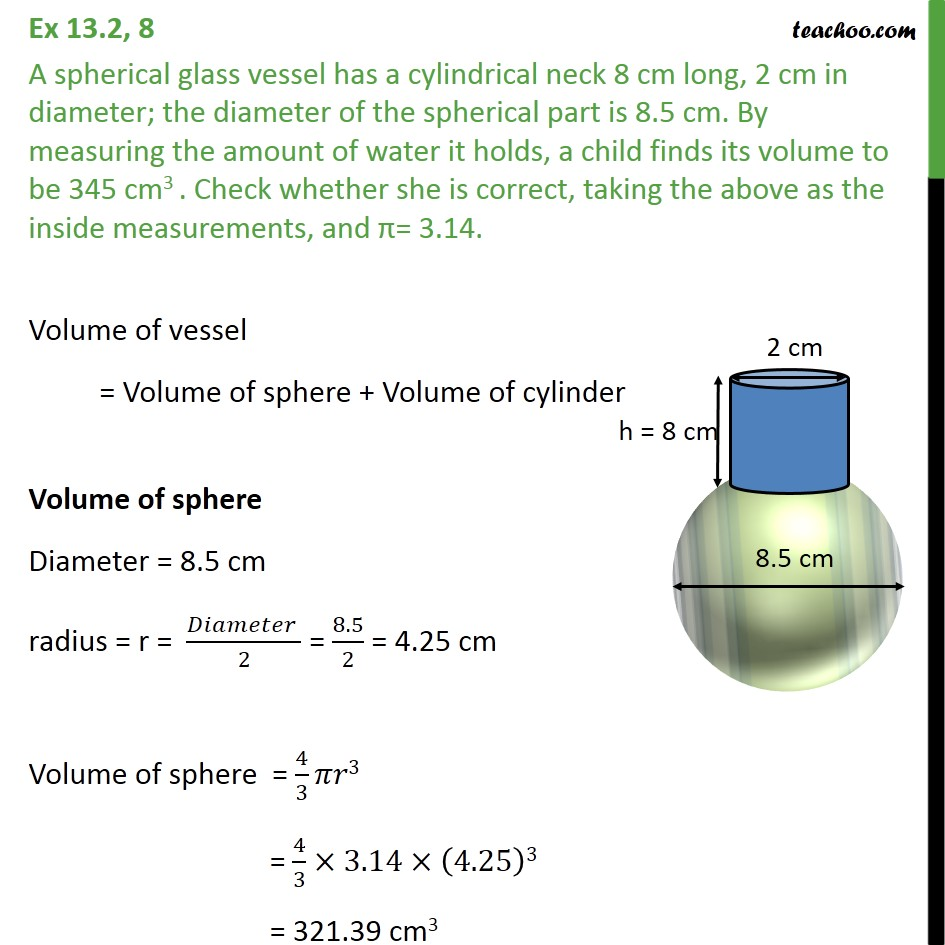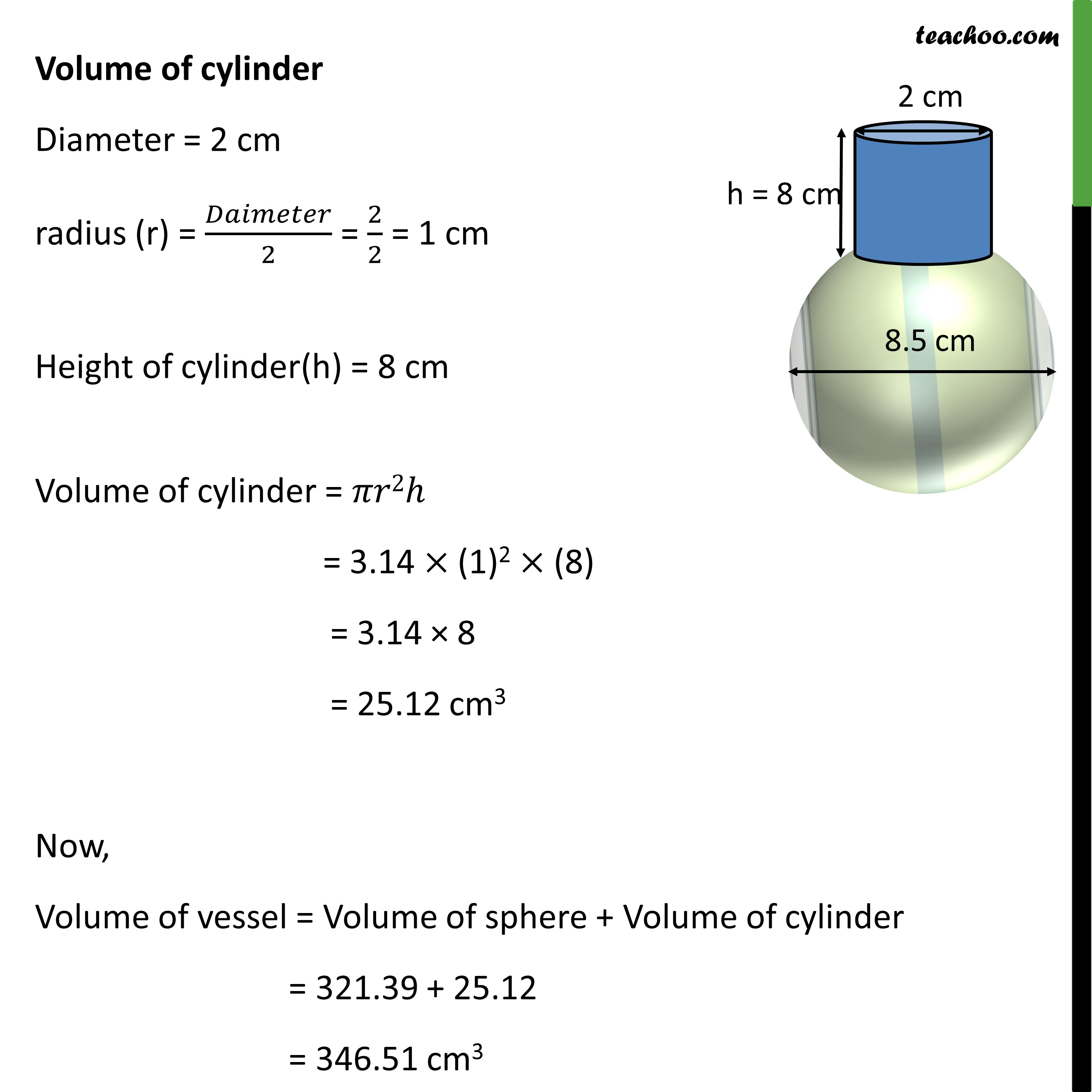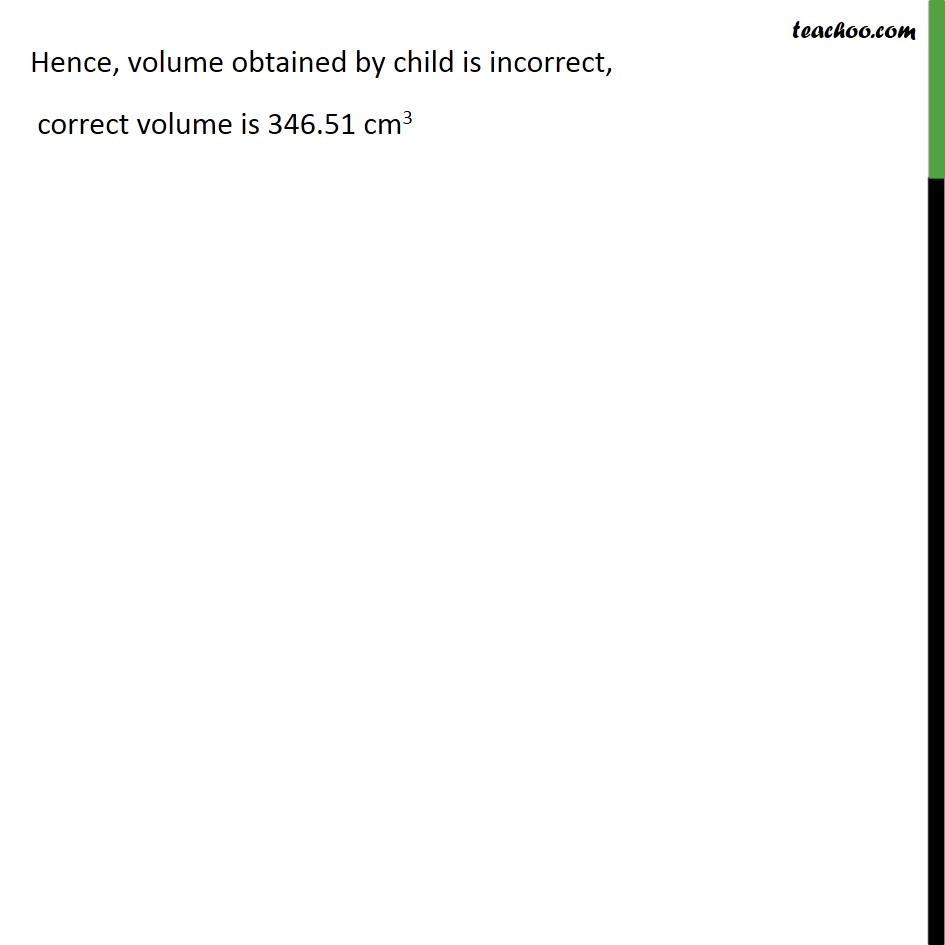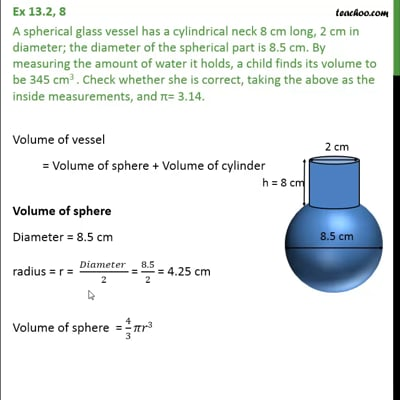Ex 13.2

Chapter 13 Class 10 Surface Areas and Volumes
Serial order wiseThis video is only available for Teachoo black users

Maths Crash Course - Live lectures + all videos + Real time Doubt solving!

### Transcript

Ex 13.2, 8 A spherical glass vessel has a cylindrical neck 8 cm long, 2 cm in diameter; the diameter of the spherical part is 8.5 cm. By measuring the amount of water it holds, a child finds its volume to be 345 cm3 . Check whether she is correct, taking the above as the inside measurements, and = 3.14. Volume of vessel = Volume of sphere + Volume of cylinder Volume of sphere Diameter = 8.5 cm radius = r = ( )/2 = 8.5/2 = 4.25 cm Volume of sphere = 4/3 3 = 4/3 3.14 (4.25)3 = 321.39 cm3 Volume of cylinder Diameter = 2 cm radius (r) = /2 = 2/2 = 1 cm Height of cylinder(h) = 8 cm Volume of cylinder = 2 = 3.14 (1)2 (8) = 3.14 8 = 12.12 cm3 Now, Volume of vessel = Volume of sphere + Volume of cylinder = 321.39 + 25.12 = 346.51 cm3 Hence, volume obtained by child is incorrect, correct volume is 346.51 cm3